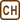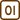## Cold Sheet

Estimate how many degrees Celsius can we reduce from the temperature of a sick child by wrapping him or her in a wet sheet.

Note: Given that human body consists mainly of water, consider that its specific heat capacity has the same value as water. Assume that 30 % of the heat the child “gives off” is lost to the surroundings. Also neglect the metabolism, i.e. the chemical reactions during which the heat is released, and which maintains the body temperature. Estimate or measure the necessary data.

• #### Solution

It is a relatively simple task which uses the calorimetric formula. We know that the water in the sheet absorbs only 70 % of the released heat. We include the specific heat capacity of the sheet in the losses to the surroundings, i.e. we consider only the heat exchange between the child and water.

We get an equation:

$0.7 Q_{\mathrm{released}} = Q_{\mathrm{absorbed}}$

$0.7 c m_c (t_c - t) = c m_w (t - t_w),$

where mc and tc are the mass and the temperature of the child, mw and tw are the mass and the temperature of water, and t is the resulting temperature.

We consider the specific heat capacity of water and the child to be the same. Thus we can reduce:

$0.7 m_c (t_c - t) = m_w (t - t_w).$

We can evaluate the unknown t from this equation. First we multiply out the parentheses. :

$0.7 m_c t_c - 0.7 m_c t = m_w t - m_w t_w,$

Then we transfer the terms containing the unknown t to the right side of the equation:

$0.7 m_c t + m_w t = 0.7 m_c t_c + m_w t_w$

Now we factor out and evaluate t:

$(0.7 m_c + m_w) t = 0.7 m_c t_c + m_w t_w$

$t =\frac{ 0.7 m_c t_c + m_w t_w}{0.7 m_c + m_w}$

We substitute the following values to the resulting relation:

– the mass of the child mc = 30 kg and his or her temperature tc = 40 °C
– the temperature of the sheet soaked with water tw = 15 °C
– the amount of the water kept in the sheet mw = 1.5 kg

$t =\frac{ 0.7 \cdot{30} \cdot{40} + 1.5 \cdot{15}}{0.7 \cdot{30} + 1.5}\,\mathrm{^\circ C} = 38\,\mathrm{^\circ C}$